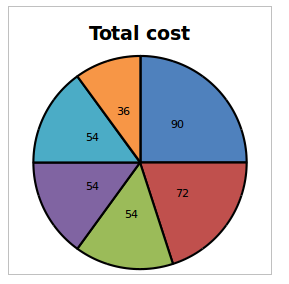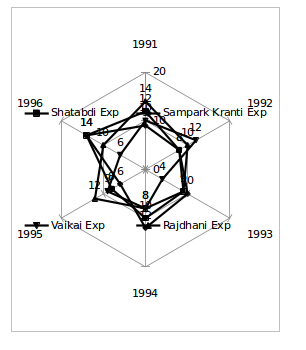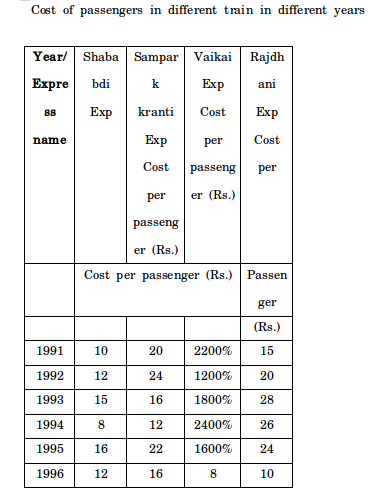## Important Data Interpretation For SBI PO : Set – 69

D.1-5) Study the following graph carefully to answer the questions:

Pie chart showing the cost of constructing one house in degree in the year 1991

Total Cost = 12 lakhsTable shows the percentage of Cost of construction increased for two years 1992-1993 from the previous year

 Year 1992 1993 Labour 12% 14% Cement 24% 16% Bricks 14% 15% Steel 25% 10% Supervisor 15% 25% Timber 10% 20%

1) what percentage the amount spent on timber in the year 1993 is the amount spent on supervisor and steel in the year 1993?

a) 43.5%

b) 31.29%

c) 34.3%

d) 43.33%

e) None of these

b)

Timber cost in 1991=12×36/360=1.2 lakh

Timber cost in 1993=1.2×110/100×120/100=1.584

Supervisor cost in 1991=12×54/360=1.8 lakh

Supervisor cost in 1993=1.8×115/100×125/100=2.5875

Steel cost in 1991=54×12/360=1.8 lakh

Steel cost in 1993=1.8×125/100×110/100=2.475

Required percentage=1.584/(2.475+2.5875)×100=31.29%

2) In what ratio sum of the amount spent on cement and labour in the year 1992 and the difference between the sum of the amount spend timber and bricks in the year 1992 and the sum of the amount spent in supervisor and steel in the year 1993? (Approximately)

a) 16:5

b) 5:16

c) 12: 5

d) 12:7

e) None of these

a)

Cement cost in 1991=72×12/360=2.4

cement cost in 1992=124/100×2.4=2.976

Labour cost in 1991=90×12/360=3

labour cost in 1992=112/100×3=3.36

bricks cost in 1991=54×12/360=1.8

bricks cost in 1992=1.8×114/100=2.05

timber cost in 1991=36×12/360=1.2

timber cost in 1992=1.2×110/100=1.32

supervisor cost in 1991=54×12/360=1.8

supervisor cost in 1993=1.8×115/100×125/100=2.5875

steel cost in 1991=54×12/360=1.8

steel cost in 1993=125/100×110/100×1.8=2.475

Required ratio~6.4 lakh :2 lakh=16:5

3) What is the difference between the sum of the cost of cements and timber in the year 1993 and the sum of the cost of steel and supervisor in the year 1993(in Rs.)?

a) 2034

b) 2676

c) 3305

d) 2634

e) None of these

d)

Cost of cement in the year 1993=(12×72)/360×124/100×116/100=345216

Cost of timper in the year 1993=(12×36)/360×110/100×120/100=158400

Cost of steel in the year 1993=(12×54)/360×(125/100110)/100=247500

Cost of supervisor in the year 1993=(12×54)/360×115/100×125/100=258750

Required difference=503616-506250=2634

4) Which one has the maximum average cost in all the three years together?

a) Cement

b) Bricks

c) Labour

d) Supervisor

e) Timber

c)

Cost of labour in the year 1991=(12×90)/360=3

Cost of labour in the year 1992=3×112/100=3.36

Cost of labour in the year 1993=3.36×114/100=3.83

Average cost of labour in all the year=(3+3.36+3.83)/3=3.397 lakh

Cost of cement in the year 1991=72×12/360=2.4

Cost of cement cost in the year 1992=124/100×2.4=2.976

Cost of cement cost in the year 1993=2.976×116/100=3.45

Average cost of cement in all the year=(2.4+2.976+3.45)/3=2.942 lakh

Cost of bricks cost in the year 1991=(12×54)/360=1.8

Cost of bricks cost in the year 1992=1.8×114/100=2.052

Cost of bricks cost in the year 1993=2.052×115/100=2.359

Average cost of bricks in all the year=(1.8+2.052+2.359)/3=2.07 lakh

Cost of supervisor in the year 1991=(12×54)/360=1.8

Cost of supervisor in the year 1992=1.8×115/100=2.07

Cost of supervisor in the year 1993=2.07×125/100=2.5875

Average cost of supervisor in all the years=(1.8+2.07+2.5875)/3=2.15 lakh

Cost of timber in the year 1991=12×36/360=1.2

Cost of timber in the year 1992=1.2×110/100=1.32

Cost of timber in the year 1993=1.32×120/100=1.584

Average cost of timber in all the year=(1.2+1.32+1.584)/3=1.368 lakh

Cost of Steel in the year 1991=12×54/360=1.8

Cost of Steel in the year 1992=1.8×125/100=2.25

Cost of Steel in the year 1993=2.25×110/100=2.475

Average cost of Steel in all the year=(1.8+2.25+2.475)/3=2.175 lakh

Average of labour has maximum cost in all the years together

5) If the cost of labour in 1994 is 20% more than the difference between the sum of the cost of bricks and labour in the year 1993 and the sum of the cost of timber and steel in the year 1993, the cost of labour in 1994 is how much percent more/less than the cost of supervisor in the year 1992?

a) 14%

b) 24%

c) 21.3%

d) 23.47

e) None of these

d)

Cost of bricks in 1993=(12×54)/360×114/100×115/100=2.35

Cost of labour in 1993=(12×90)/360×112/100×114/100=3.83

Cost of timber in 1993=(12×36)/360×110/100×120/100=1.58

Cost of steel in 1993=(12×54)/360×125/100×110/100=2.475

Required difference=6.19-4.0595=2.13

Supervisor cost in 1992=(12×54)/360×115/100=2.07

labour cost in 1994=120/100×2.13=2.556

Required percentage=(2.556-2.07)/2.07×100=23.47%

D.6-10) Study the following graph carefully to answer the questions:

The number of passengers travelled (in hundreds) in four different trains in different years6) What is the difference between the total of the cost of Vaikai express in the year 1991, 1992, 1993 and 1994 and the total of the cost of Rajdhani express in the year 1993, 1994, 1995 and 1996?

a) 24000

b) 20000

c) 22000

d) 32000

e) None of these

c)

Total cost of vaikai express=(1000×22)+(1200×12)+(400×18)+(800+24)

=62800

Total cost of Rajdhani express=(900×28)+(800×26)+(1200×24) (1000×10)=84800

Required difference=84800-62800=22000

7) In the year 1997, all the trains cost per passengers increased by 20% of the previous year and the number of passengers same in the previous year, total cost of all the express in the year 1997 is how much percentage more/less cost of all the express in 1995?

a) 5.35%

b) 6.7%

c) 6.5%

d) 6.35%

e) None of these

d)

In the year 1997 cost per passenger in sababdi exp=120/100×12=14.4

In the year 1997 cost per passenger in sampark kranti exp=120/100×16=19.2

In the year 1997 cost per passenger in vaikai exp=120/100×8=9.6

In the year 1997 cost per passenger in Rajdhani exp=120/100×10=12

Total cost of all the express in the year 1997

=(14.4×1400)+(1400×19.2)+(600×9.6)+(12×1000)

=20160+26880+5760+12000=64800

Total cost of all the express in the year 1995

=(16×800)+(600×22)+(900×16)+(24×1200)

=12800+13200+14400+28800=69200

Required percentage=(64800-69200)/69200×100=6.35%

8) If the number of passengers 30% increased in the year 1992 in all the express, Shababdi express cost in the year 1993 is 20% more than the difference between the total cost of Vaikai and Samparkkranti express in the year 1994 and the total cost of Rajdhani express in the year 1993, 1994 and 1995, approximately what percentage of Shababdi express cost in the year 1993 is total cost of Vaikai express in the year 1992 after the increment?

a) 317%

b) 320%

c) 318%

d) 321%

e) None of these

a)

sababdi express cost in 1993

=120/100×((800×24+1200×12)-(900×28+800×26+1200×24))=49440

Number of passenger in the year 1992 in vaikai exp=1000×130/100=1300

Total cost in vaikai express after increment=1300×12=15600

Required percentage=49440/15600×100~317%

9) In which year has the maximum cost of average per passenger in all the express together?

a) 1993

b) 1995

c) 1992

d) 1996

e) 1994

b)

Average cost in the year 1991=(10×1200+20×900+22×1000+1400×15)/4500=16.22

Average cost in the year 1992=(12×800+24×800+12×1200+20×1000)/3800=16.63

Average cost in the year 1993=(15×900+16×1000+18×400+28×900)/3200=19.34

Average cost in the year 1994=(8×1000+12×1200+24×800+26×800)/3800=16.42

Average cost in the year 1995=(16×800+22×600+16×900+24×1200)/3500=19.77

Average cost in the year 1996=(12×1400+16×1400+8×600+10×1000)/4400=12.27

In the year 1995 average cost is maximum.

10) In what amount the total cost of Shababdi express in the year 1992, 1994 and 1996 and the difference between the total cost of Vaikai in the year 1993, 1995, and 1996 and the total cost of Samparkkranti in the year 1991, 1992 and 1993?

a) 86180

b) 26400

c) 87000

d) 61200

e) None of these